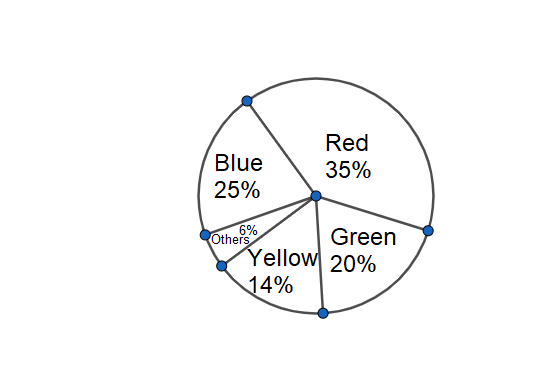Courses
Courses for Kids
Free study material
Offline Centres
MoreLast updated date: 06th Dec 2023
Total views: 282k
Views today: 8.82k

# Students of a class voted for their favourite colour according to the below pie chart. Observe it and find the appropriate option.A. $\dfrac{1}{20}th$ student voted for blue colour.B. Green is the least popular colour.C. The number of students who voted for blue colour is two times the number of students who voted for yellow colour.D. Number of students liking yellow and green colours is approximately the same as those of red colour.Verified
282k+ views
Hint: We assume the total number of students voting and also the individual number of students for each colour. We then verify the given statements in the options to find the correct one.

Complete step-by-step solution:
Let us assume the total students’ number is $100x$.
The respective percentages for red, blue, green, yellow and others are 35, 25, 20, 14 and 6 respectively.
We know for any arbitrary percentage value of a%, we can write it as $\dfrac{a}{100}$. The percentage is to find the respective value out of 100.
Therefore, the number for students voting for colour red is $100x\times \dfrac{35}{100}=35x$.
Similarly, the number for students voting for colour blue, green, yellow and others are $100x\times \dfrac{25}{100}=25x$, $100x\times \dfrac{20}{100}=20x$, $100x\times \dfrac{14}{100}=14x$, $100x\times \dfrac{6}{100}=6x$ respectively.
The fraction for colour blue is $\dfrac{25x}{100x}=\dfrac{1}{4}$. The first option is wrong.
Yellow has less students voting for it than green and that’s why it can’t be the least popular colour.
Two times the number of students who voted for yellow colour will be $14x\times 2=28x$ which is not equal to the number of students who voted for blue colour.
Number of students liking yellow and green colours is $20x+14x=34x$ which is approximately the same as those of red colour.
The correct option is D.

Note: We can also use the total percentage as the base of the calculation. The total percentage gives $35+25+20+14+6=100$. That covers the whole circle which helps in getting the fractional value.